A popular Internet meme these days is to present an arithmetic expression like, say, 6/3(4−2) and ask the poor souls who follow you to decide the right answer. Soon there will be two camps, each convinced that they know the truth and that the others are illiterate fools: According to one camp, the answer is 4, whereas the other camp will swear that it has to be 1.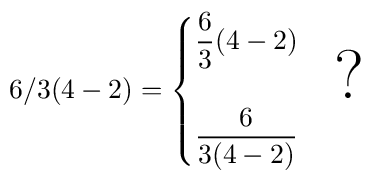In reality it is neither. Or both. Flip a coin, take your pick. There is no fundamental mathematical truth hidden here. It all boils down to human conventions. The standard convention is that multiplication and division have the same precedence and are evaluated from left to right: So 6/3×(4−2) is pretty unambiguous. But there is another, unwritten convention that when the multiplication sign is omitted, the implied multiplication is assumed to have a higher precedence.

Precisely because of these ambiguities, when you see actual professionals, mathematicians or physicists, write down an expression like this, they opt for clarity: they write, say, (6/3)(4−2) or 6/[3(4−2)] precisely so as to avoid any misunderstanding. Or better yet, they use proper math typesetting software such as LaTeX and write 2D formulas.I met Gabor David back in 1982 when I became a member of the team we informally named F451 (inspired by Ray Bradbury of course.) Gabor was a close friend of Ferenc Szatmari. Together, they played an instrumental role in establishing a business relationship between the Hungarian firm Novotrade and its British partner, Andromeda, developing game programs for the Commodore 64.

In the months and years that followed, we spent a lot of time working together. I was proud to enjoy Gabor’s friendship. He was very knowledgeable, and also very committed to our success. We had some stressful times, to be sure, but also a lot of fun, frantic days (and many nights!) spent working together.

I remember Gabor’s deep, loud voice, with a slight speech impediment, a mild case of rhotacism. His face, too, I can recall with almost movie like quality.

He loved coffee more than I thought possible. He once dropped by at my place, not long after I managed to destroy my coffee maker, a stovetop espresso that I accidentally left on the stove for a good half hour. Gabor entered with the words, “Kids, do you have any coffee?” I tried to explain to him that the devil’s brew in that carafe was a bitter, undrinkable (and likely unhealthy) blend of burnt coffee and burnt rubber, but to no avail: he gulped it down like it was nectar.

After I left Hungary in 1986, we remained in sporadic contact. In fact, Gabor helped me with a small loan during my initial few weeks on Austria; for this, I was very grateful.

When I first visited Hungary as a newly minted Canadian citizen, after the collapse of communism there, Gabor was one of the few close friends that I sought out. I was hugely impressed. Gabor was now heading a company called Banknet, an international joint venture bringing business grade satellite-based Internet service to the country.

When our friend Ferenc was diagnosed with lung cancer, Gabor was distraught. He tried to help Feri with financing an unconventional treatment not covered by insurance. I pitched in, too. It was not enough to save Feri’s life: he passed away shortly thereafter, a loss I still feel more than two decades later.

My last conversation with Gabor was distressing. I don’t really remember the details, but I did learn that he suffered a stroke, and that he was worried that he would be placed under some form of guardianship. Soon thereafter, I lost touch; his phone number, as I recall, was disconnected and Gabor vanished.

Every so often, I looked for him on the Internet, on social media, but to no avail. His name is not uncommon, and moreover, as his last name also doubles as a first name for many, searches bring up far too many false positives. But last night, it occurred to me to search for his name and his original profession: “Dávid Gábor” “matematikus” (mathematician).

Jackpot, if it can be called that. One of the first hits that came up was a page from Hungary’s John von Neumann Computer Society, their information technology history forum, to be specific: a short biography of Gabor, together with his picture.

And from this page I learned that Gabor passed away almost six years ago, on November 10, 2014, at the age of 72.

Well… at least I now know. It has been a privilege knowing you, Gabor, and being able to count you among my friends. I learned a lot from you, and I cherish all those times that we spent working together.

I am one of the maintainers of the Maxima computer algebra system. Maxima’s origins date back to the 1960s, when I was still in kindergarten. I feel very privileged that I can participate in the continuing development of one of the oldest continuously maintained software system in wide use.

It has been a while since I last dug deep into the core of the Maxima system. My LISP skills are admittedly a bit rusty. But a recent change to a core Maxima capability, its ability to create Taylor-series expansions of expressions, broke an important feature of Maxima’s tensor algebra packages, so it needed fixing.

The fix doesn’t amount to much, just a few lines of code: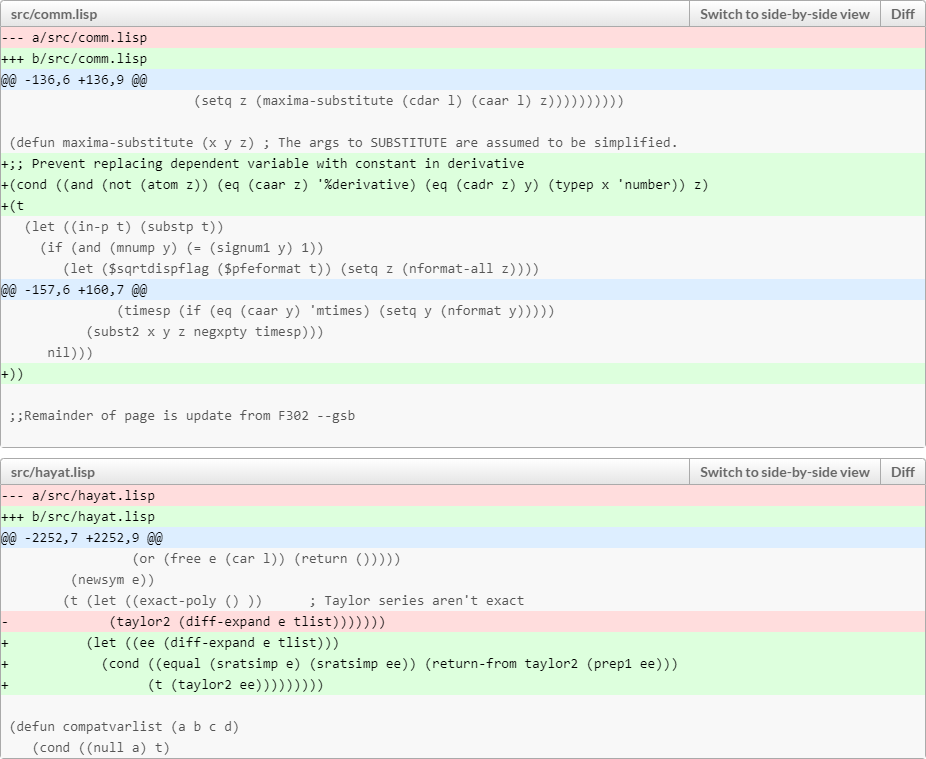It did take more than a few minutes though to find the right (I hope) way to implement this fix.

Even so, I had fun. This is the kind of programming that I really, really enjoy doing. Sadly, it’s not the kind of programming for which people usually pay you Big Bucks… Oh well. The fun alone was worth it.

So here I am, reading about some trivial yet not-so-trivial probability distributions.

Let’s start with the uniform distribution. Easy-peasy, isn’t it: a random number, between 0 and 1, with an equal probability assigned to any value within this range.

So… what happens if I take two such random numbers and add them? Why, I get a random number between 0 and 2 of course. But the probability distribution will no longer be uniform. There are more ways to get a value in the vicinity of 1 than near 0 or 2.

And what happens if I add three such random numbers? Or four? And so on?

The statistics of this result are captured by the Irwin-Hall distribution, defined as

$$f_{\rm IH}(x,n)=\dfrac{1}{2(n-1)!}\sum\limits_{k=1}^n(-1)^k\begin{pmatrix}n\\k\end{pmatrix}(x-k)^{n-1}{\rm sgn}(x-k).$$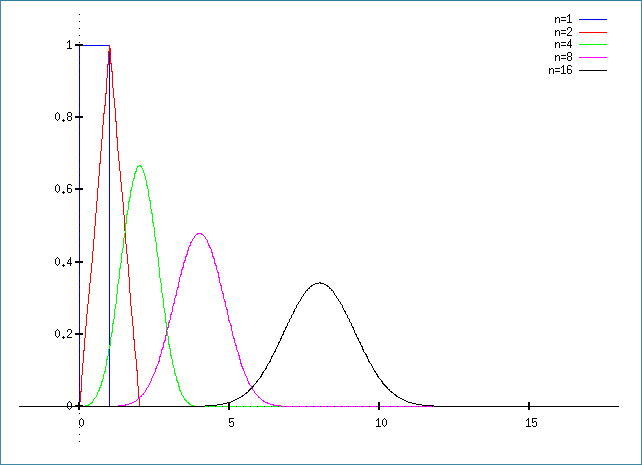OK, so that’s what happens when we add these uniformly generated random values. What happens when we average them? This, in turn, is captured by the Bates distribution, which, unsurprisingly, is just the Irwin-Hall distribution, scaled by the factor $$n$$:

$$f_{\rm B}(x,n)=\dfrac{n}{2(n-1)!}\sum\limits_{k=1}^n(-1)^k\begin{pmatrix}n\\k\end{pmatrix}(nx-k)^{n-1}{\rm sgn}(nx-k).$$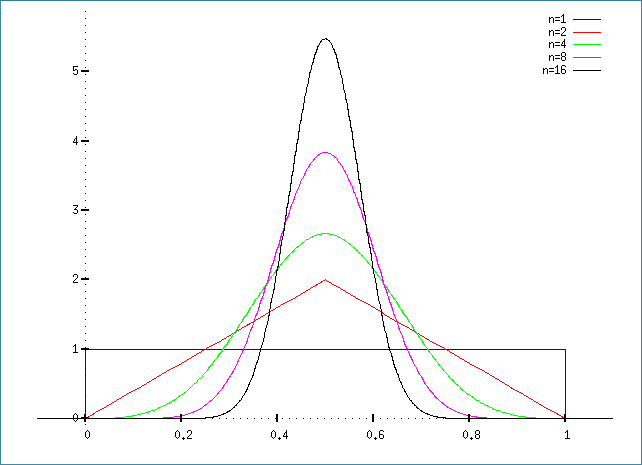For what it’s worth, here is the Maxima script to generate the Irwin-Hall plot:

fI(x,n):=1/2/(n-1)!*sum((-1)^k*n!/k!/(n-k)!*(x-k)^(n-1)*signum(x-k),k,0,n);
plot2d([fI(x,1),fI(x,2),fI(x,4),fI(x,8),fI(x,16)],[x,-2,18],[box,false],
[legend,"n=1","n=2","n=4","n=8","n=16"],[y,-0.1,1.1]);

And this one for the Bates plot:

fB(x,n):=n/2/(n-1)!*sum((-1)^k*n!/k!/(n-k)!*(n*x-k)^(n-1)*signum(n*x-k),k,0,n);
plot2d([fB(x,1),fB(x,2),fB(x,4),fB(x,8),fB(x,16)],[x,-0.1,1.1],[box,false],
[legend,"n=1","n=2","n=4","n=8","n=16"],[y,-0.1,5.9]);

Yes, I am still a little bit of a math geek at heart.

My lovely wife, Ildiko, woke up from a dream and asked: If you have a flower with 7 petals and two colors, how many ways can you color the petals of that flower?Intriguing, isn’t it.

Such a flower shape obviously has rotational symmetry. Just because the flower is rotated by several times a seventh of a revolution, the resulting pattern should not be counted as distinct. So it is not simply calculating what number theorists call the $$n$$-tuple. It is something more subtle.

We can, of course, start counting the possibilities the brute force way. It’s not that difficult for a smaller number of petals, but it does get a little confusing at 6. At 7 petals, it is still something that can be done, but the use of paper-and-pencil is strongly recommended.

So what about the more general case? What if I have $$n$$ petals and $$k$$ colors?

Neither of us could easily deduce an answer, so I went to search the available online literature. For a while, other than finding some interesting posts about cyclic, or circular permutations, I was mostly unsuccessful. In fact, I began to wonder if this one was perhaps one of those embarrassing little problems in combinatorial mathematics that has no known solution and about which the literature remains strangely quiet.

But then I had another idea: By this time, we both calculated the sequence, 2, 3, 4, 6, 8, 14, 20, which is the number of ways flowers with 1, 2, …, 7 petals can be colored using only two colors. Surely, this sequence is known to Google?

Indeed it is. It turns out to be a well-known sequence in the online encyclopedia of integer sequences, A000031. Now I was getting somewhere! What was especially helpful is that the encyclopedia mentioned necklaces. So that’s what this problem set is called! Finding the Mathworld page on necklaces was now easy, along with the corresponding Wikipedia page. I also found an attempt, valiant though only half-successful if anyone is interested in my opinion, to explain the intuition behind this known result:

$$N_k(n)=\frac{1}{n}\sum_{d|n}\phi(d)k^{n/d},$$

where the summation is over all the divisors of $$n$$, and $$\phi(d)$$ is Euler’s totient function, the number of integers between $$1$$ and $$d$$ that are relative prime to $$d$$.

Evil stuff if you asked me. Much as I always liked mathematics, number theory was not my favorite.

In the case of odd primes, such as the number 7 that occurred in Ildiko’s dream, and only two colors, there is, however, a simplified form:

$$N_2(n)=\frac{2^{n-1}-1}{n}+2^{(n-1)/2}+1.$$

Substituting $$n=7$$, we indeed get 20.

Finally, a closely related sequence, A000029, characterizes necklaces that can be turned over, that is to say, the case where we do not count mirror images separately.

Oh, this was fun. It’s not like I didn’t have anything useful to do with my time, but it was nonetheless a delightful distraction. And a good thing to chat about while we were eating a wonderful lunch that Ildiko prepared today.

And before I forget: Last week, wearing my release manager hat I successfully created a new version of Maxima, the open-source computer algebra system. As a result, Maxima is again named one of SourceForge’s projects of the week, for the week of June 10.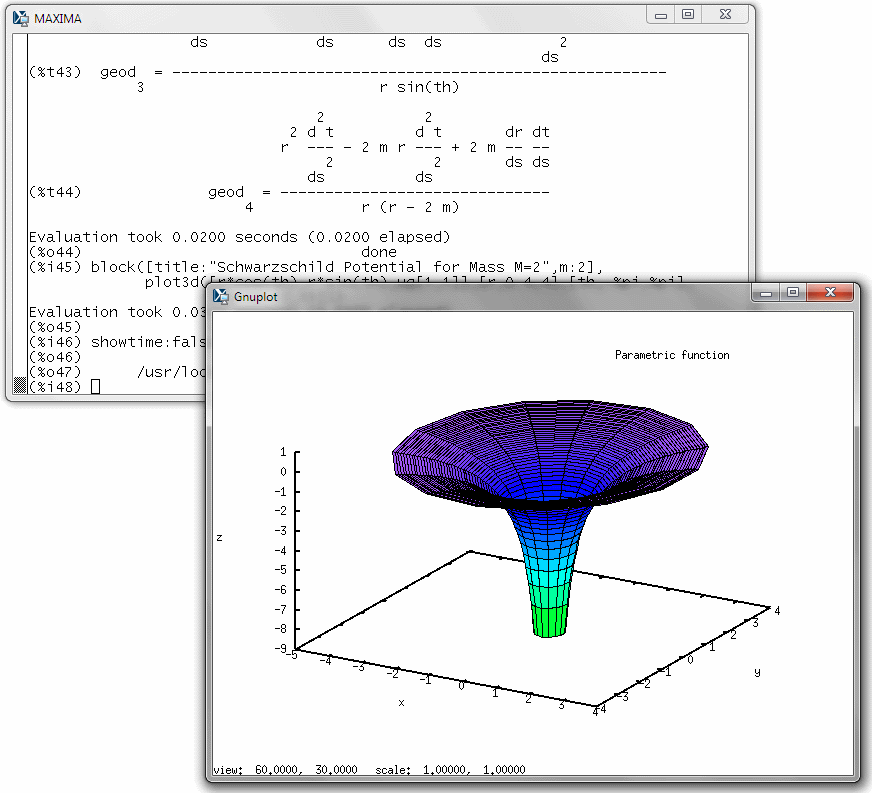The release turned out to be more of an uphill battle than I anticipated, but in the end, I think everything went glitch-free.

Others have since created installers for different platforms, including Windows.

And I keep promising myself that when I grow up, I will one day understand exactly what git does and how it works, instead of just blindly following arcane scripts…

Years ago, I accepted the role of release manager for the Maxima computer algebra system. It proved to be more laborious than I assumed (mostly for two things: assembling changelogs, and dealing with build glitches) but it still has its upside. Right now, it is my pleasure to announce that Maxima 5.42 has been released on the unsuspecting public. Enjoy!Michael Atiyah, 89, is one of the greatest living mathematicians. Which is why the world pays attention when he claims to have solved what is perhaps the greatest outstanding problem in mathematics, the Riemann hypothesis.

Here is a simple sum: $$1+\frac{1}{2^2}+\frac{1}{3^2}+…$$. It is actually convergent: The result is $$\pi^2/6$$.

Other, similar sums also converge, so long as the exponent is greater than 1. In fact, we can define a function:

\begin{align*}\zeta(x)=\sum\limits_{i=1}^\infty\frac{1}{i^x}.\end{align*}

Where things get really interesting is when we extend the definition of this $$\zeta(x)$$ to the entire complex plane. As it turns out, its analytic continuation is defined almost everywhere. And, it has a few zeros, i.e., values of $$x$$ for which $$\zeta(x)=0$$.

The so-called trivial zeros of $$\zeta(x)$$ are the negative even integers: $$x=-2,-4,-6,…$$. But the function also has infinitely many nontrivial zeros, where $$x$$ is complex. And here is the thing: The real part of all known nontrivial zeros happens to be $$\frac{1}{2}$$, the first one being at $$x=\frac{1}{2}+14.1347251417347i$$. This, then, is the Riemann hypothesis: Namely that if $$x$$ is a non-trivial zero of $$\zeta(x)$$, then $$\Re(x)=\frac{1}{2}$$. This hypothesis baffled mathematicians for the past 130 years, and now Atiyah claims to have solved it, accidentally (!), in a mere five pages. Unfortunately, verifying his proof is above my pay grade, as it references other concepts that I would have to learn first. But it is understandable why the mathematical community is skeptical (to say the least).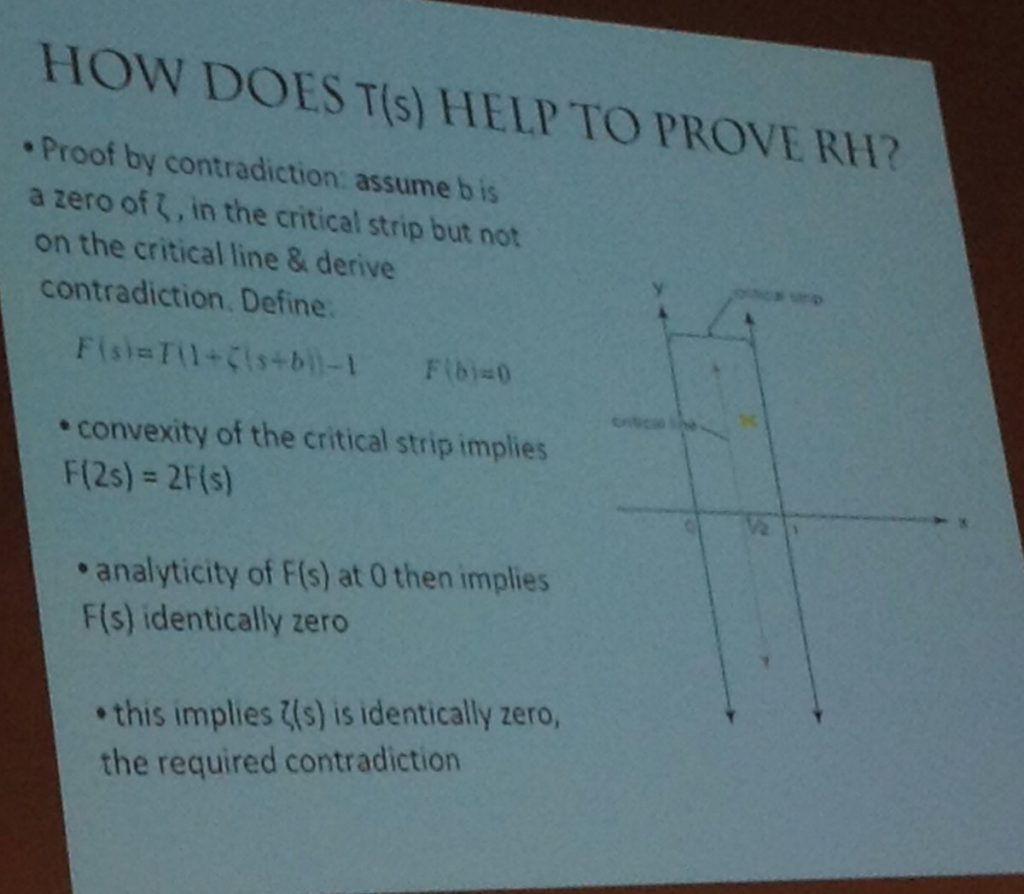A slide from Atiyah’s talk on September 24, 2018.

What is not above my pay grade is analyzing Atiyah’s other claim: a purported mathematical definition of the fine structure constant $$\alpha$$. The modern definition of $$\alpha$$ relates this number to the electron charge $$e$$: $$\alpha=e^2/4\pi\epsilon_0\hbar c$$, where $$\epsilon_0$$ is the electric permeability of the vacuum, $$\hbar$$ is the reduced Planck constant and $$c$$ is the speed of light. Back in the days of Arthur Eddington, it seemed that $$\alpha\sim 1/136$$, which led Eddington himself onto a futile quest of numerology, trying to concoct a reason why $$136$$ is a special number. Today, we know the value of $$\alpha$$ a little better: $$\alpha^{-1}\simeq 137.0359992$$.

Atiyah produced a long and somewhat rambling paper that fundamentally boils down to two equations. First, he defines a new mathematical constant, denoted by the Cyrillic letter $$\unicode{x427}$$ (Che), which is related to the fine structure constant by the equation

\begin{align*}\alpha^{-1}=\frac{\pi\unicode{x427}}{\gamma},\tag{1.1*}\end{align*}

where $$\gamma=0.577…$$ is the Euler–Mascheroni constant. Second, he offers a definition for $$\unicode{x427}$$:

\begin{align*}\unicode{x427}=\frac{1}{2}\sum\limits_{j=1}^\infty 2^{-j}\left(1-\int_{1/j}^j\log_2 x~dx\right).\tag{7.1*}\end{align*}

(The equation numbers are Atiyah’s; I used a star to signify that I slightly simplified them.)

Atiyah claims that this sum is difficult to calculate and then goes into a long-winded and not very well explained derivation. But the sum is not difficult to calculate. In fact, I can calculate it with ease as the definite integral under the summation sign is trivial:

\begin{align*}\int_{1/j}^j\log_2 x~dx=\frac{(j^2+1)\log j-j^2+1}{j\log 2}.\end{align*}

After this, the sum rapidly converges, as this little bit of Maxima code demonstrates (NB: for $$j=1$$ the integral is trivial as the integration limits collapse):

(%i1) assume(j>1);
(%o1)                               [j > 1]
(%i2) S:1/2*2^(-j)*(1-integrate(log(x)/log(2),x,1/j,j));
log(j) + 1
---------- + j log(j) - j
(- j) - 1          j
(%o2)             2          (1 - -------------------------)
log(2)
(%i3) float(sum(S,j,1,50));
(%o3)                         0.02944508691740671
(%i4) float(sum(S,j,1,100));
(%o4)                         0.02944508691730876
(%i5) float(sum(S,j,1,150));
(%o5)                         0.02944508691730876
(%i6) float(sum(S,j,1,100)*%pi/%gamma);
(%o6)                         0.1602598029967022


Unfortunately, this does not look like $$\alpha^{-1}=137.0359992$$ at all. Not even remotely.

So we are all left to guess, sadly, what Atiyah was thinking when he offered this proposal.

We must also remember that $$\alpha$$ is a so-called “running” constant, as its value depends on the energy of the interaction, though presumably, the constant in question here is $$\alpha$$ in the infrared limit, i.e., at zero energy.

Enough of politics and cats. Time to blog about math and physics again.

Back in my high school days, when I was becoming familiar with calculus and differential equations (yes, I was a math geek) something troubled me. Why were certain expressions called “linear” when they obviously weren’t?

I mean, an expression like $$Ax+B$$ is obviously linear. But who in his right mind would call something like $$x^3y + 3e^xy+5$$ “linear”? Yet when it comes to differential equations, they’d tell you that $$x^3y+3e^xy+5-y^{\prime\prime}=0$$ is “obviously” a second-order, linear ordinary differential equation (ODE). What gives? And why is, say, $$xy^3+3e^xy-y^{\prime\prime}=0$$ not considered linear?

The answer is quite simple, actually, but for some reason when I was 14 or so, it took a very long time for me to understand.

Here is the recipe. Take an equation like $$x^3y+3e^xy+5-y^{\prime\prime}=0$$. Throw away the inhomogeneous bit, leaving the $$x^3y+3e^xy-y^{\prime\prime}=0$$ part. Apart from the fact that it is solved (obviously) by $$y=0$$, there is another thing that you can discern immediately. If $$y_1$$ and $$y_2$$ are both solutions, then so is their linear combination $$\alpha y_1+\beta y_2$$ (with $$\alpha$$ and $$\beta$$ constants), which you can see by simple substitution, as it yields $$\alpha(x^3y_1+3e^xy_1-y_1^{\prime\prime}) + \beta(x^3y_2+3e^xy_2-y_2^{\prime\prime})$$ for the left-hand side, with both terms obviously zero if $$y_1$$ and $$y_2$$ are indeed solutions.

So never mind that it contains higher derivatives. Never mind that it contains powers, even transcendental functions of the independent variable $$x$$. What matters is that the expression is linear in the dependent variable. As such, the linear combination of any two solutions of the homogeneous equation is also a solution.

Better yet, when it comes to the solutions of inhomogeneous equations, adding a solution of the homogeneous equation to any one of them yields another solution of the inhomogeneous equation.

Notably in physics, the Schrödinger equation of quantum mechanics is an example of a homogeneous and linear differential equation. This becomes a fundamental aspect of quantum physics: given two solutions (representing two distinct physical states) their linear combination is also a solution, representing another possible physical state.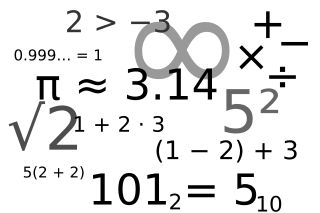I am watching the morning news and it’s all about numbers. Some good, some not so good, some really bad. Here are a few, in descending order:

• 2018: The year when Ottawa plans to introduce a new low-income transit fare.
• 417: The provincial highway number of the Queensway, which has been reopened after yesterday’s huge crash.
• 175.6: The amount of rain, in mm, that Ottawa received in the month of May.
• 80: The estimated number killed by a massive ISIS terrorist bomb in Kabul.
• 21: The highest expected temperature of the day and, incidentally, the entire week, in Centigrade.
• 15: The new minimum wage, in Canadian dollars, as proposed by the Ontario provincial government.
• 7: The age of a baby, in months, who died allegedly due to her mother’s negligence in Gatineau.

I thought of turning these bullet points into a numbered list, but that would have been too confusing.

I have been so busy this week, I forgot to blog about our latest Maxima release, 5.39. Nothing spectacular, just incremental improvements over 5.38; for me, this was a big milestone though as this was the first time that I used a CentOS platform to prepare the release. (Which, incidentally, is why I haven’t done this months ago.)And SourceForge, kindly enough, once again designated Maxima as one of the site’s Projects of the Week.

Sometime last year, I foolishly volunteered to manage new releases of the Maxima computer algebra system (CAS).

For the past several weeks, I’ve been promising to do my first release, but I kept putting it off as I had other, more pressing work obligations.

Well, not anymore… today, I finally found the time, after brushing up on the Git version management system, and managed to put together a release, 5.38.0.Maxima is beautiful and incredibly powerful. I have been working on its tensor algebra packages for the past 15 years or so. As far as I know, Maxima is the only general purpose CAS that can derive the field equations of a Lagrangian field theory; for instance, it can derive Einstein’s field equations from the Einstein-Hilbert Lagrangian.

I use Maxima a lot for tensor algebra, though I admit that when it comes to integration, differential equations or plotting, I prefer Maple. Maple’s ODE/PDE solvers are unbeatable. But when it comes to tensor algebra, or just as a generic on-screen symbolic calculator, Maxima wins hands down. I prefer to use its command-line version: Nothing fancy, just ASCII art, but very snappy, very responsive, and does exactly what I want it to do.

So then, Maxima 5.38.0: Say hi to the world. World, this is the latest version of the oldest (nearly half a century old) continuously maintained CAS in existence.

Having just finished work on a major project milestone, I took it easy for a few days, allowing myself to spend time thinking about other things. That’s when I encountered an absolutely neat problem on Quora.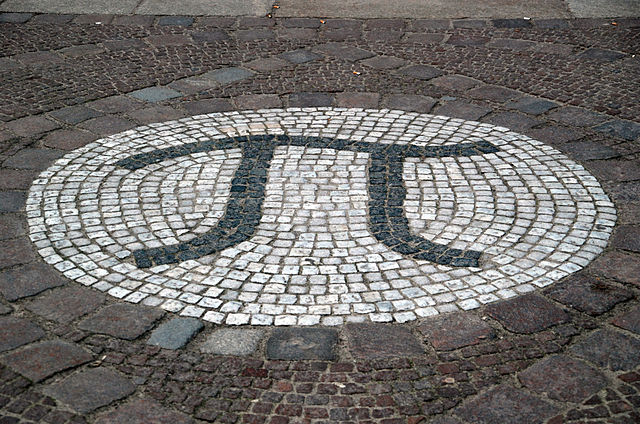Someone asked a seemingly innocuous number theory question: are there two positive integers such that one is exactly the π-th power of the other?

Now wait a minute, you ask… We know that π is a transcendental number. How can an integer raised to a transcendental power be another integer?

But then you think about $$\alpha=\log_2 3$$ and realize that although $$\alpha$$ is a transcendental number, $$2^\alpha=3$$. So why can’t we have $$n^\pi=m$$, then?

As it turns out, we (probably) cannot, but the reason is subtle and it relies on a very important, but unproven conjecture from transcendental number theory.

But first, let us rewrite the equation by taking its logarithm:

$$\pi\log n = \log m.$$

We can also divide both sides by $$\log n$$, which leads to

$$\pi = \frac{\log m}{\log n}=\log_n m,$$

but it turns out to be not very helpful. However, squaring the equation will help, as we shall shortly see:

$$\pi^2\log^2 n=\log^2 m.$$

Can this equation ever by true for positive integers $$n$$ and $$m$$, other than the trivial solution $$n=m=1$$, that is?

To see why it cannot be the case, let us consider the following triplet of numbers:

$$(i\pi,\log n,\log m),$$

and their exponents,

$$(e^{i\pi}=-1, e^{\log n}=n, e^{\log m}=m).$$

The three numbers $$(i\pi,\log n,\log m)$$ are linearly independent over $${\mathbb Q}$$ (that is, the rational numbers). What this means is that there are no rational numbers $$A, B, C, D$$ such that $$Ai\pi+B\log n+C\log m + D=0$$. This is easy to see as the ratio of $$\log n$$ and $$\log m$$ is supposed to be transcendental but both numbers are real, whereas $$i\pi$$ is imaginary.

On the other hand, their exponents are all rational numbers ($$-1, n, m$$). And this is where the unproven conjecture, Schanuel’s conjecture, comes into the picture. Schanuel’s conjecture says that given $$n$$ complex numbers $$(\alpha_1,\alpha_2,…,\alpha_n)$$ that are linearly independent over the rationals, out of the $$2n$$ numbers $$(\alpha_1,…,\alpha_n,e^{\alpha_1},…,e^{\alpha_n})$$, at least $$n$$ will be transcendental numbers that are algebraically independent over $${\mathbb Q}$$. That is, there is no algebraic expression involving roots and powers of the $$\alpha_i$$, $$e^{\alpha_i}$$, and rational numbers that will yield 0.

The equation $$\pi^2\log^2 n=\log^2 m$$, which we can rewrite as

$$(i\pi)^2\log^2 n + \log^2 m=0,$$

is just such an equation, and it can never be true.

I wish I could say that I came up with this solution but I didn’t. I was this close: I was trying to apply Schanuel’s conjecture, and I was of course using the fact that $$\pi=-i\log -1$$. But I did not fully appreciate the implications and meaning of Schanuel’s conjecture, so I was applying it improperly. Fortunately, another Quora user saved the day.

Still I haven’t had this much fun with pure math (and I haven’t learned this much pure math all at once) in years.

John Forbes Nash Jr. is dead, along with his wife Alicia. They were killed on the New Jersey Turnpike when the taxi, taking them home from the airport, crashed into a guardrail and another vehicle after the driver lost control while trying to pass.Nash and his wife were returning from Norway, where Nash was one of the recipients of the 2015 Abel prize.

News of this accident made me shudder for another reason. Less than two weeks ago, when I was returning from Dubai, my taxi driver not only answered a call on his cell phone, he even responded to a text while driving. I was too tired to say anything at first and then thankfully he came to his senses… but his behavior made me feel decidedly uncomfortable in his vehicle. Next time, I will not hesitate to tell the taxi driver to stop immediately or call another taxi for me.

Science fiction has a subgenre: mathematical fiction. Stories of this nature are rare; good stories are even rarer. One memorable story that I recall from ages ago was A Subway Named Moebius, written by A. J. Deutsch in 1950. There was another story more recently: Luminous by Greg Egan, which I read in Asimov’s SF magazine shortly before I stopped reading (and eventually, stopped subscribing to) said magazine. (Nothing wrong with the magazine; it’s just that I found many of the stories unsatisfying, and I found I had less and less time to read them. The genre is just not the same as it was back in the Golden Age of Science Fiction.)

So recently, I found out that Egan wrote a sequel: Dark Integers, published in the same magazine in 2007. I now had a chance to read it and I was not disappointed.Both stories are very good. Both stories are based on the notion that as yet unproven mathematical theorems can go either way; that the Platonic book of all math has not only not yet been written, but that there is no unique book, and multiple versions of mathematics may coexist, with an uneasy boundary.

Now imagine that you perform innocent mathematical experiments on your computer, using, say, computer algebra to probe ever more exotic theorems in a subfield few non-mathematicians ever heard about. And imagine how you would feel if you realized that by doing so, you are undermining the very foundations of another universe’s existence, literally threatening to wipe them out.

OK, if you start poking holes in that idea, there are many, but the basic notion is not completely stupid, and the questions that the stories raise are worth contemplating. And Egan writes well… the stories are fun, too!

Incidentally, this was the first decent (published) science fiction story I ever came across that contained a few lines of C++ code.

So the other day, I solved this curious mathematics puzzle using repeated applications of Pythagoras’s theorem and a little bit of algebra.

Now I realize that there is a much simpler form of the proof.

The exercise was to prove that, given two semicircles drawn into a bigger circle as shown below, the sum of the areas of the semicircles is exactly half that of the larger circle.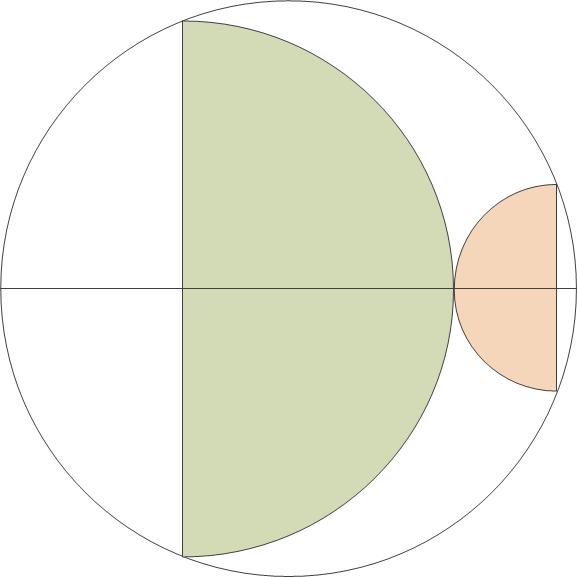Again, I’m inserting a few blank lines before presenting my proof.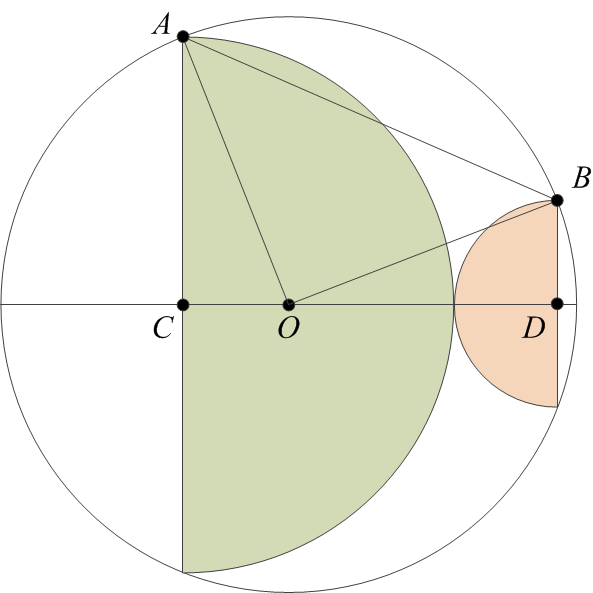Once again I am labeling some vertices in the diagram for easy reference.

Our goal is to prove that the area of a circle with radius AO is twice the sum of the areas of two semicircles, with radii AC and BD. But that is the same as proving that the area of a circle with radius AO is equal to the sum of the areas of two circles, with radii AC and BD.

The ACO< angle is a right angle. Therefore, the area of a circle with radius AO is the sum of the areas of circles with radii AC and CO. (To see this, just multiply the theorem of Pythagoras by π.) So if only we could prove that CO = BD, our proof would be complete.

Since AO = BO, they are the sides of the isosceles triangle ABO. Now if we were to pick a point O on the line CD such that CO‘ = BD, the ACO and ODB triangles will be identical (CD being the sum of AC and BD by construction). Therefore, AO‘ = BO, and the ABO triangle would be another isosceles triangle with its third vertex on the CD line. Clearly that is not possible, so O = O, and therefore, CO = BD. This concludes the proof.

The other day, I ran across a cute geometry puzzle on John Baez’s Google+ page. I was able to solve it in a few minutes, before I read the full post that suggested that this was, after all, a harder-than-usual area puzzle. Glad to see that, even though the last high school mathematics competition in which I participated was something like 35 years ago, I have not yet lost the skill.

Anyhow, the puzzle is this: prove that the area of the two semicircles below is exactly half the area of the full circle.I am going to insert a few blank lines here before providing my solution.

I start with labeling some vertices on the diagram and also drawing a few radii and other lines to help.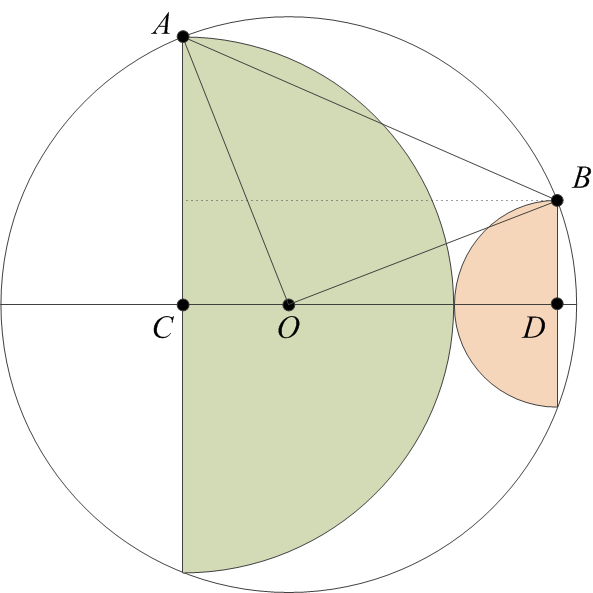Next, let’s call the radii of the two semicircles as $$a$$ and $$b$$. Then, we have
\begin{align}
(AC)&= a,\\
(BD)&= b.
\end{align}Now observe that
\begin{align}
(OA) = (OB) = r,
\end{align}and also
\begin{align}
(CD)&= a + b,\\
(OD)&= a + b~- (OC).
\end{align}The rest is just repeated application of the theorem of Pythagoras:
\begin{align}
(OC)^2&= r^2 – a^2,\\
(OD)^2&= r^2 – b^2,
\end{align}followed by a bit of trivial algebra:
\begin{align}
(OC)^2 + a^2&= [a + b – (OC)]^2 + b^2,\\
0&= 2(a + b)[b – (OC)],\\
(OC)&= b.
\end{align}Therefore,
\begin{align}
a^2+b^2=r^2,
\end{align}which means that the area of the full circle is twice the sum of the areas of the two semicircles, which is what we set out to prove.

I guess I have not yet lost my passion for pointless, self-serving mathematics.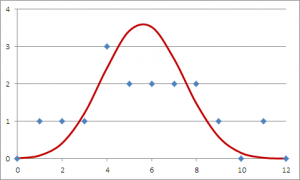Reader’s Digest recently conducted an interesting experiment: they “lost” 12 wallets, filled with about \$50 worth of cash and sufficient documentation to locate the owner, in 16 cities around the world. The result: Finns in Helsinki are the most honest with 11 of the 12 wallets returned, whereas in Lisbon, Portugal, the sole wallet that was returned was, in fact, found by a visiting Dutch couple. Finns needless to say, are rejoicing: “we don’t even run red lights,” boasted a Helsinki resident.

So what can we conclude from this interesting experiment? Perhaps shockingly, almost nothing.

This becomes evident if I plot a histogram with the number of wallets returned, and overlay on it a binomial distribution for a probability of 46.875% (which corresponds to the total number of wallets returned, 90 out of 192), I get a curve that is matched very closely by the histogram. Unsurprisingly, there will be a certain probability that in a given city, 1, 2, 3, etc. wallets are returned; and the results of Reader’s Digest match this prediction closely.

So there is no reason for Finns to rejoice or for the Portuguese to feel shame. It’s all just blind luck, after all. And the only valid conclusion we can draw from this experiment is that people are just as likely to be decent folks in Lisbon as in Helsinki.

But how do you explain this to a lay audience? More importantly, how do you prevent a political demagogue from drawing false or unwarranted conclusions from the data?

It is now formally official: global surface temperatures did not increase significantly in the past 15 years or so.

But if skeptics conclude that this is it, the smoking gun that proves that all climate science is hogwash, they better think again. When we look closely, the plots reveal something a lot more interesting.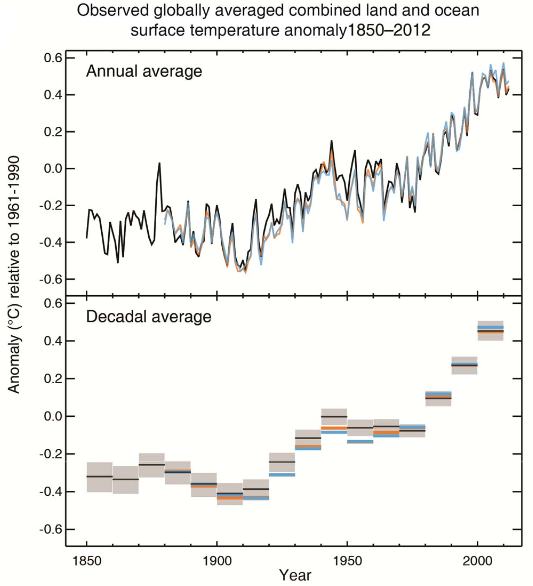For starters… this is not the first time global temperatures stagnated or even decreased somewhat since the start of recordkeeping. There is a roughly 20-year period centered around 1950 or so, and another, even longer period centered roughly around 1890. This looks in fact like evidence that there may be something to the idea of a 60-year climate cycle. However, the alarming bit is this: every time the cycle peaks, temperatures are higher than in the previous cycle.

The just released IPCC Summary for Policymakers makes no mention of this cycle but it does offer an explanation for the observed stagnating temperatures. These are probably a result of volcanic activity, they tell us, the solar cycle, and perhaps mismodeling the effects of greenhouse gases and aerosols, but they are not exactly sure.

And certainty is characterized with words like “high confidence,” “medium confidence” and such, with no definitions given. These will be supplied, supposedly, in the technical report that will be released on Monday. Nonetheless, the statement that “Probabilistic estimates […] are based on statistical analysis of observations or model results, or both, and expert judgment” [emphasis mine] does not fill me with confidence, if you will pardon the pun.

In fact, I feel compelled to compare this to the various reports and releases issued by the LHC in recent years about the Higgs boson. There was no “expert judgment”. There were objective statistical analysis methods and procedures that were thoroughly documented (even though they were often difficult to comprehend, due to their sheer complexity.) There were objective standards for claiming a discovery.

Given the extreme political sensitivity of the topic, I think the IPCC should adopt similar or even more stringent standards of analysis as the LHC. Do away with “expert judgment” and use instead proper statistical tools to establish the likelihood of specific climate models in the light of the gathered data. And if the models do not work, e.g., if they failed to predict stagnating temperatures, the right thing to do is say that this is so; there is no need for “expert judgment”. Just state the facts.